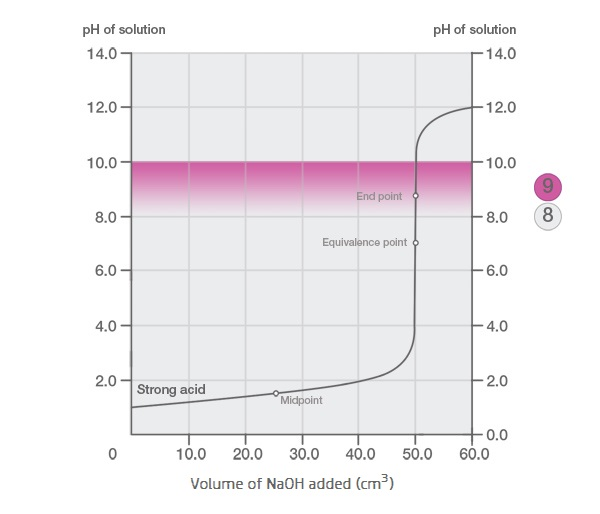An equivalence point is the theoretical completion of the reaction. It means the number of moles of base is equal to the number of moles of acid. The equivalence point is determined stoichiometrically.

Example:

NaOH(aq) + HCl(aq) → NaCl(aq) + H2O(l)

If the reaction has a 1:1 mole ratio, the number of moles of alkali is equal to the number of moles of acid. The pH of the solution when the reaction of NaOH and HCL reach equivalence point is 7.Figure: Titration curve. This curve shows the increase of pH level during the titration of a strong acid solution with sodium hydroxide (NaOH). The equivalence point is reached at pH 7. The endpoint is detected at pH 8.2.

Read more about the end-point which is often confused with the equivalence point.